### 产品中心

#### 135-2302-0101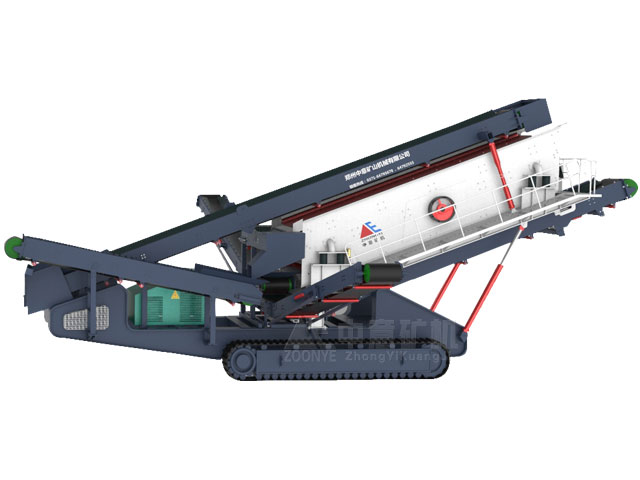# LDSF系列履带式移动筛分站

#### 客户案例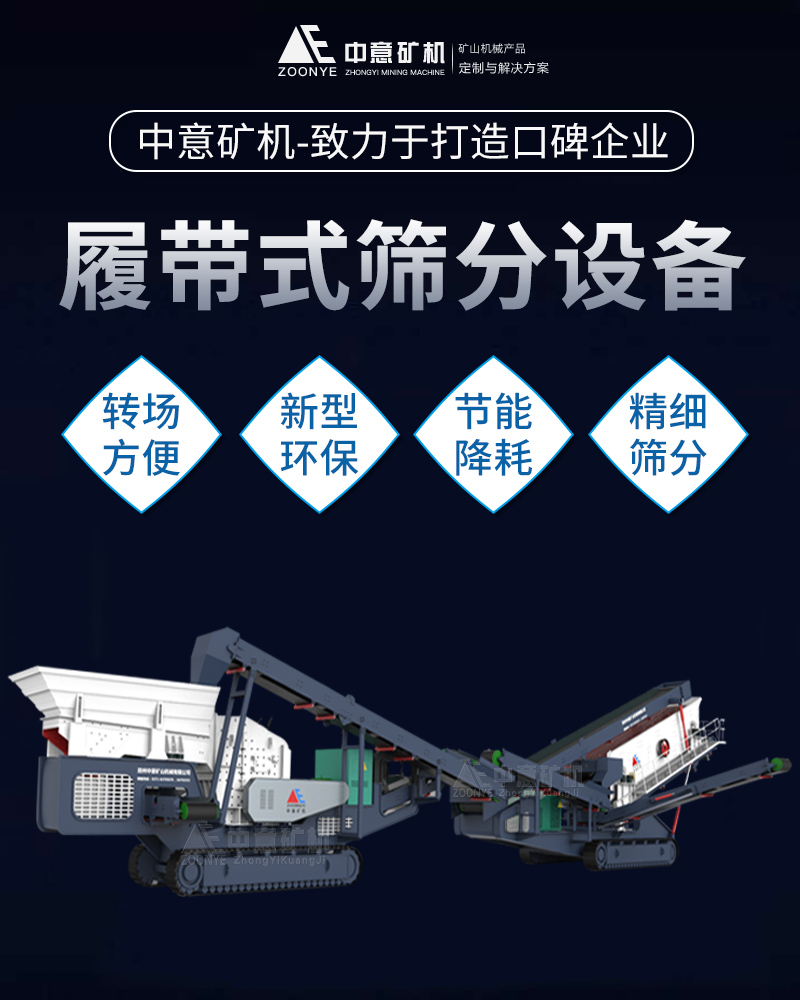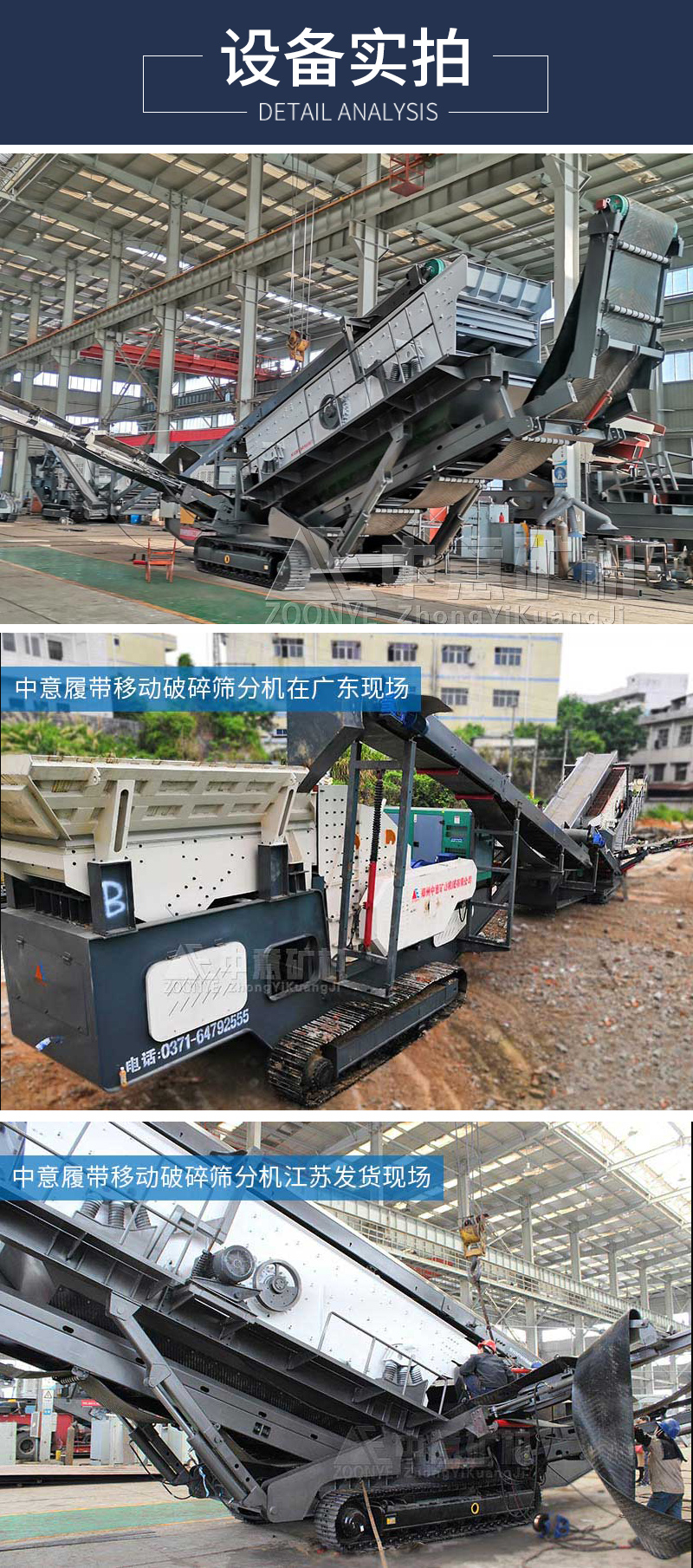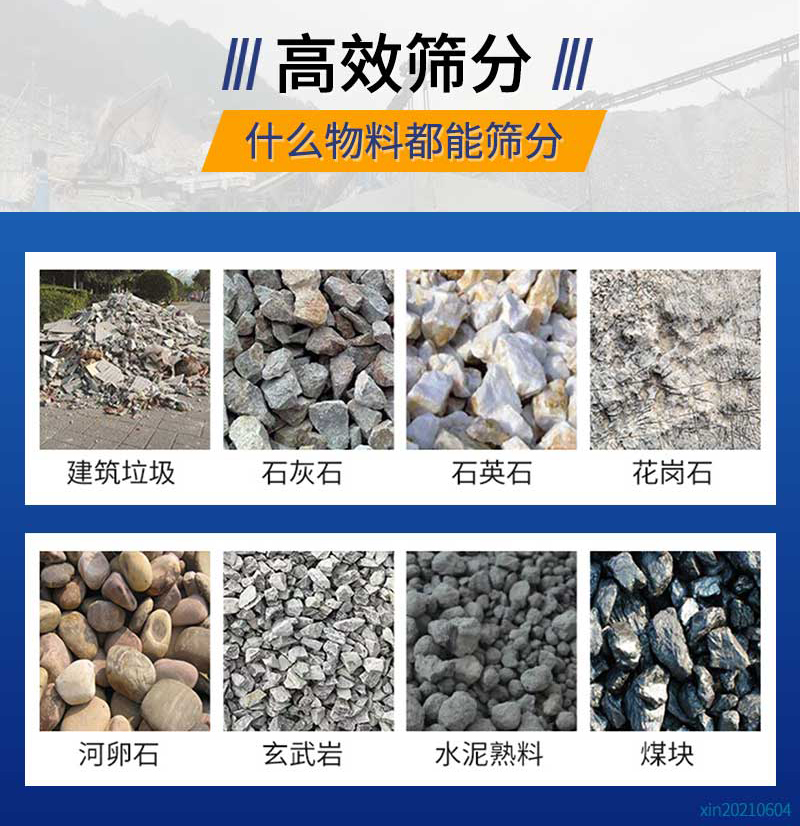### 产品优势：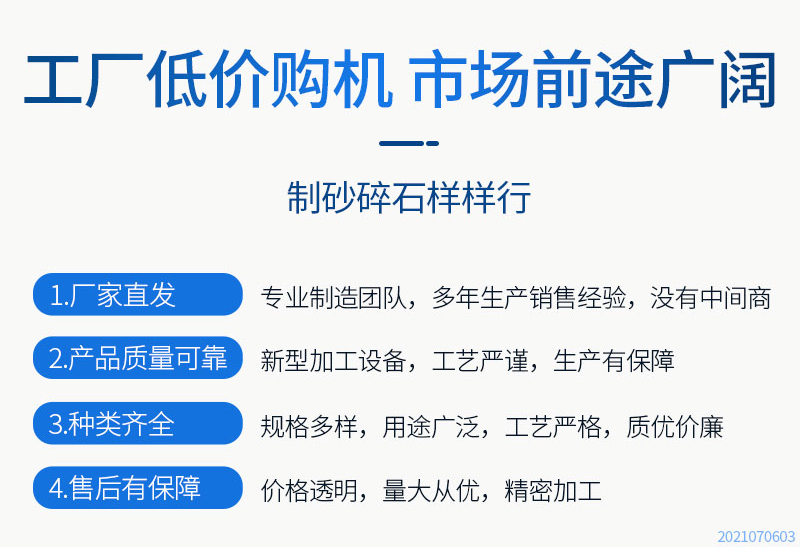1、产量大、筛净率高，生产成本低

2、振动平稳、工作可靠、寿命长

3、结构简单、重量轻、体积小、便于维护保养

### 工作原理：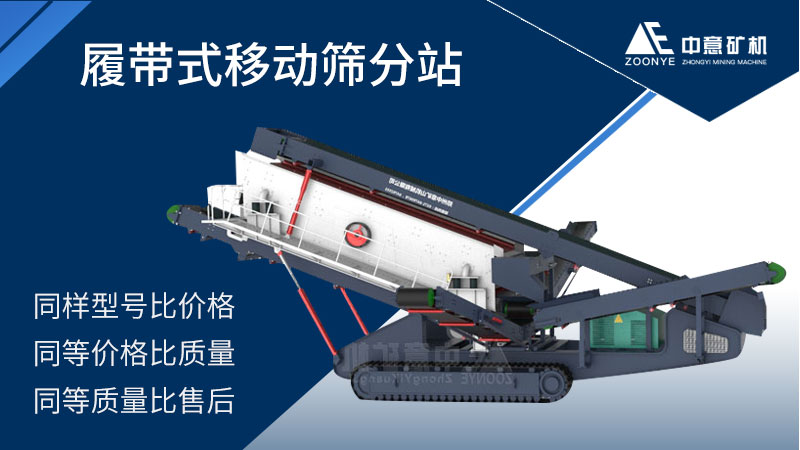### 技术参数：

 原料仓 给料皮带机 振动筛 船型钢架 履带总成 柴油机组（潍坊） 液压总成 电器总成 钢结构件 皮带运输机（筛下） 皮带运输机（返料） 皮带运输机（车架上 皮带运输机（成品） 皮带运输机（出料） 总功率（kw） LDSF1860 LDYDS B800=12.3m 3YKX1860 LDYDS LDYDS-26T 145GF LDYDS LDYDS LDYDS B1000=8.6m B500=5.7m B650=2.1m B500=6.4m 61KW LDSF2160 LDYDS B1000=12.3m 3YA2160 LDYDS LDYDS-26T 145GF-C-D LDYDS LDYDS LDYDS B1200=8.6m B650=5.7m B650=2.1m B650=6.4m 76.5KW

### 技术参数：

 原料仓 给料皮带机 振动筛 船型钢架 履带总成 柴油机组（潍坊） 液压总成 电器总成 钢结构件 皮带运输机（筛下） 皮带运输机（返料） 皮带运输机（车架上 皮带运输机（成品） 皮带运输机（出料） 总功率（kw） LDSF1860 LDYDS B800=12.3m 3YKX1860 LDYDS LDYDS-26T 145GF LDYDS LDYDS LDYDS B1000=8.6m B500=5.7m B650=2.1m B500=6.4m 61KW LDSF2160 LDYDS B1000=12.3m 3YA2160 LDYDS LDYDS-26T 145GF-C-D LDYDS LDYDS LDYDS B1200=8.6m B650=5.7m B650=2.1m B650=6.4m 76.5KW
•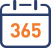#### 365天

随时为您服务

•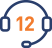#### 12小时

内快速售后响应

•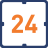#### 24小时

内现场解决故障

•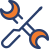享受成本价维修